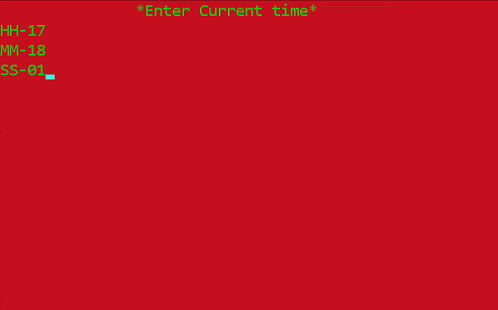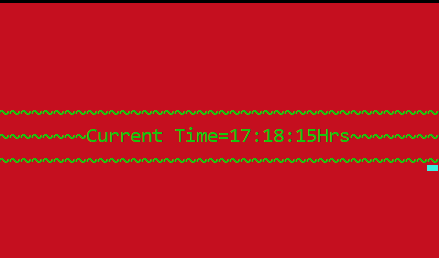Related Articles

# Digital Clock starting from user set time in C++

• Difficulty Level : Easy
• Last Updated : 25 Mar, 2021

In this article, we will discuss the Digital Clock in C++ Language. It is an application that allows for a personal clock that starts at a custom set time and shows the time from that point onwards. This article describes how to make such a clock in a 24-hour format with HH:MM:SS slots and start the time from where one would want it to be, and then it moves forward from there.

Features: It is a Simple Digital clock developed using basic C++ concepts that shows hour, minute, and second.

Approach: The requirements for this program are just the basic concepts of the data types, variables, manipulators, Control statements, Conditional statements, etc. Below are the steps:

• Create a screen that will show “Current time” of your location which will be implemented using simple output methods used in C++ i.e., cout, and a manipulator “setw()“.
• In the fore mentioned screen, implement the HH column, MM column, SS column, that will contain the time.
• Implement colors using System(“color 4A”), the color will be in hexadecimal format and the console can be used to implement them using double-digit hex codes (0 to F) which will, in turn, change the text color in the console of the output.
• In the last screen, a digital clock can be seen finally implemented and running from the inputted time.

Functions Used:

• System(“cls”): It is used to clear the Console or the Screen. It can be avoided if anyone wants to see whatever is appearing on the screen.
• setw(): This function is used to leave the space of particular characters that you can write in the parenthesis. It is declared in <iomanip> header file. Here setw(70) is used.
• System(“color 4A”): This function is used to make background RED and text as LIGHT GREEN.
• Sleep(): sleep is a function that is declared in <windows.h> header file. It actually suspends the execution of the program temporarily for a period of time in milliseconds.

Below is the implementation of the above approach:

## C++

 `// C++ program to illustrate the digital``// clock starting from the entered time`` ` `#include ``#include ``#include ``#include ``using` `namespace` `std;`` ` `// Driver Code``int` `main()``{``    ``system``(``"color 4A"``);`` ` `    ``// Background color and Foreground``    ``int` `hour, min, sec;``    ``cout << setw(70)``         ``<< ``"*Enter Current time*\n"``;`` ` `    ``// Use of manipulator for taking``    ``// input from the user``    ``cout << ``"HH- "``;``    ``cin >> hour;``    ``cout << ``"MM- "``;``    ``cin >> min;``    ``cout << ``"SS- "``;``    ``cin >> sec;`` ` `    ``// Background color and the``    ``// Foreground for 2nd screen``    ``system``(``"color 4A"``);`` ` `    ``// Cases for the Wrong Time Input``    ``if` `(hour > 23) {``        ``cout << ``"Wrong Time input"``;``    ``}``    ``else` `if` `(min > 60) {``        ``cout << ``"Wrong Time Input"``;``    ``}``    ``else` `if` `(sec > 60) {``        ``cout << ``"Wrong Time Input"``;``    ``}`` ` `    ``// Otherwise``    ``else` `{``        ``while` `(1)`` ` `        ``// Run Block infinitely``        ``{``            ``system``(``"cls"``);`` ` `            ``// Clear the console`` ` `            ``// Code for Showing Time``            ``for` `(hour; hour < 24; hour++) {`` ` `                ``for` `(min; min < 60; min++) {`` ` `                    ``for` `(sec; sec < 60; sec++) {``                        ``system``(``"cls"``);`` ` `                        ``cout << ``"\n\n\n\n~~~~~~~~~"``                                ``"~~~~~~~~~~~~~~~~~~~~~"``                                ``"~~~~~~~~~~~~~~~~~~"``                                ``"Current Time = "``                             ``<< hour << ``":"` `<< min << ``":"``                             ``<< sec``                             ``<< ``"Hrs~~~~~~~~~~~~~~~~~~"``                                ``"~~~~~~~~~~~~~~~~~~~~~"``                                ``"~~~~~~~~~"``;`` ` `                        ``// HH:MM:SS columns in output`` ` `                        ``Sleep(1000);`` ` `                        ``// Pause for 1 sec``                    ``}``                    ``sec = 0;``                ``}``                ``min = 0;``            ``}``        ``}``    ``}``}`

Input:Output:Want to learn from the best curated videos and practice problems, check out the C++ Foundation Course for Basic to Advanced C++ and C++ STL Course for foundation plus STL.  To complete your preparation from learning a language to DS Algo and many more,  please refer Complete Interview Preparation Course.

My Personal Notes arrow_drop_up